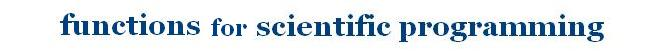You are working on isPrime functionsearchContents: swap function: Swapping two values to change their addresses. a max function: The largest number among two. a absolute value function: The positive value of a number. a sorting function: How to sort an array. a bisection function: Bissection method used to solve equations by computing, example of cable strung .. a Newton-Raphson function: To solve equations numerically, example of free fall motion .. a Secant function: To solve equations numerically, example of free fall motion .. a isPrime function: This function tests whether an integer is prime.. a abundant function: This function tests whether an integer is abundant.. a factorial function: Factorial of an integer.. a quadratic function: solving a quadratic equation .. a even_odd function: To know if an integer is even or odd .. a mean value function: To calculate the arithmetic mean of some values.. a reverse function: To reverse an integer and to know whether a string is palindrome.. a least squares function : To calculate the slope and the y_intercept of a linear equation .. a Monte Carlo method: To calculate integrales or probabilities using Monte Carlo technique .. a 2 and 3 equations systems solving : To solve the 2 and 3 equations systems with 2 and 3 variables .. a Interpolation and Extrapolation : To solve for lacking values in graphs or tables .. a Integer divisors : Gives the divisors fo an integer and tests whether it is prime .. a Amicable pair : Gives, if it exists, the amicable number of a given integer .. a Perfect numbers : Tests whether a positive integer is perfect, and more .. a Periodic table of elements: Periodic table of elements in C language, and more .. a Playing TicTacToe Using some Fortran90 language funtionalities, and more .. a a home

 @import url(http://www.google.com/cse/api/branding.css);Custom Search © 2007. The scientificsentence . All rights reserved.